# Logical Reasoning - Test 3

## 25 Questions MCQ Test SSC CGL Tier 1 Mock Test Series | Logical Reasoning - Test 3

Description
Attempt Logical Reasoning - Test 3 | 25 questions in 15 minutes | Mock test for SSC preparation | Free important questions MCQ to study SSC CGL Tier 1 Mock Test Series for SSC Exam | Download free PDF with solutions
QUESTION: 1

### Directions: In the following question, select the related number from the given alternatives. 45 : 60 ∷ 120 : ?

Solution:

In 45 : 60
45 = 9 × 5
60 = 10 × 6
Similarly;
120 = 12 × 10
143 = 13 × 11
Hence, “143” is correct option.

QUESTION: 2

### In the following question, select the related letters from the given alternatives. FEGH : IJLK ∷ NMOP : ?

Solution:

In FEGH : IJLK;
F + 3 = I; E + 5 = J; G + 5 = L;H + 3 = K
Similarly;
N + 3 = Q; M + 5 = R; O + 5 = T; P + 3 = S
Hence,

QUESTION: 3

### In the following question, select the related word from the given alternatives. Chemist : Lab :: Students : ?

Solution:

As chemist carries out his research in the lab likewise students study in the class.

QUESTION: 4

Select the odd word from the given responses.

Solution:

Dictionary, Novel and Journal is a type of book but Book itself can be Novel, Dictionary and Journal.
Hence, among all Book is odd one out.

QUESTION: 5

Select the odd number from the given responses.

Solution:

1. 5431 = 5 – 4 = 1; 4 – 3 = 1; 3 – 1 = 2
2. 9875 = 9 – 8 = 1; 8 – 7 = 1; 7 – 5 = 2
3. 7653 = 7 – 6 = 1; 6 – 5 = 1; 5 – 3 = 2
4. 6547 = 6 – 5 = 1; 5 – 4 = 1; 4 – 7 = -3
Here we can see that difference of last two digit is 2 in every option except d where the difference is 3.
Hence option “6547” is different from other responses

QUESTION: 6

In the following questions, find the odd one out from the given alternatives.

Solution:

Here the pattern followed is that each letter is increased by 2 than the previous one.

1. YZBDF = Y + 1 = Z;         Z + 2 = B;         B + 2 = D;        D + 2 = F

2. QSUWY = Q + 2 = S;         S + 2 = U;         U + 2 = W;       W + 2 = Y

3. TVXZB = T + 2 = V;         V + 2 = X;         X + 2 = Z;         Z + 2 = B

4. IKMOQ = I + 2 = K;          K + 2 = M;        M + 2 = O;       O + 2 = Q

In YZBDF, Z is only one more than Y hence it doesn’t follow the pattern and hence it is odd one out

QUESTION: 7

Which one set of letters when sequentially placed at the gaps in the given letter series shall complete the given series?

_n m m n _n m n n _m n m n_

Solution:
1. nn m m n nn m n n mm n m n= doesn’t form a pattern
2. nn m m n mn m n n nm n m n= doesn’t form a pattern
3. mn m m n nn m n n nm n m n= doesn’t form a pattern
4. nn m m / n mn m / n n mm / n m n= forms a perfect pattern

Hence, “n m m m” will complete the series.

QUESTION: 8

A series is given with one term missing. Select the correct alternative from the given ones that will complete the series.

1, 1, 2, 12, ?

Solution:

The followed pattern is:
First number = 1
(1 × 3) – 2 = 1
(1 × 6) – 4 = 2
(2 × 9) – 6 = 12
(12 × 12) – 8 = 136
Hence, 136 is the missing term.

QUESTION: 9

In a certain code the following numbers are coded in a certain way be assigning signs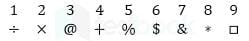Q. Which number can be decoded from the following?&%+□÷

Solution: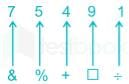Hence, “&%+□÷ “ will be coded as “75491”

QUESTION: 10

If BE QUICK is coded as ZC OSGAI, then the code of last letter of third word in the sentence I LOVE MY COUNTRY is

Solution:

Here,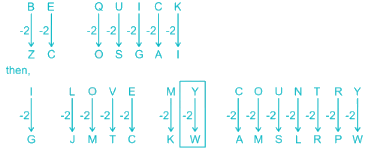Third Word is = MY.
Last letter of third word will be Y
Hence, “Y” will be coded as “W

QUESTION: 11

A boy is facing East, then he turns left and goes 12 m, then turns right and goes 6 m, then goes 6 m to the South and from there 6 m to West. In which direction is he from his original place?

Solution:

Boy is facing east and turns left i.eNorth .His path can be traced as:-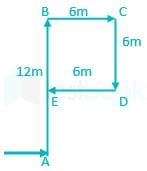A is the starting point and E is the ending point.
clearly, he is standing at north From the starting place A.

QUESTION: 12

Arrange the following words in a dictionary order.

1. Pertinent
2. Persistent
3. Peracetate
4. Permanent
5. Perborate

Solution:

After arranging as per the order of dictionary we get;
Peracetate, Perborate, Permanent, Persistent, Pertinent
Hence the correct order is 3, 5, 4, 2, 1.

QUESTION: 13

Directions: Select the odd letter form the given responses.

Solution:

1) ADBF = A + 3 = D; D – 2 = B; B + 4 = F
2) BECG = B + 3 = E; E – 2 = C;C + 4 = G
3) DHEI = D + 4 = H; H – 3 = E;E + 4 = I
4) MPNR = M + 3 = P; P - 2 = N; N + 4 = R
Hence, “DHEI” is different from other responses.

QUESTION: 14

A word given in capital letters is followed by four words. Out of these only one cannot be formed by using the letters of the given word. Find out that word.

PRESTIGIOUS

Solution:

1. PRESS = can be formed as PRESTIGIOUS.
2. PIOUS = can be formed as PRESTIGIOUS.
3. TIGER = can be formed as PRESTIGIOUS.
4. RIGID = cannot be formed as D is not present.
Hence “RIGID” is the correct option

QUESTION: 15

In the following venn diagram, identify the politicians who are corrupt and cricket player.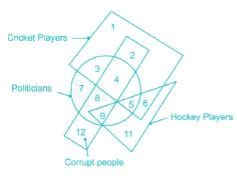Solution: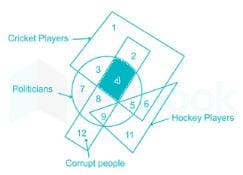Circle represents Politicians. Rectangle represents Corrupt people. Triangle represents Hockey players and Square represents Cricket players. Number which comes under circle square and rectangle represents politicians who are corrupt and cricket player which is equal to 4.

QUESTION: 16

Directions: In each of the following questions, one/two statement/statement is/are given followed by two Assumptions/Conclusions I and II. You have to consider the statement to be true, even if it seems to be at variance from commonly known facts. You are to decide which of the given assumptions/conclusions can definitely be drawn from the given statement.

Statements:
All cars are bikes.
All bikes are ships.

Conclusions:
I. All cars are ships.
II. All ships are bikes.

Solution:

representing the statements as Venn diagram:-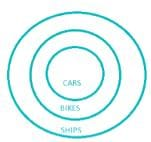I. All cars are ships = it definitely follows
II. All ships are bikes= it doesn’t follow since some ships are not bikes.
Hence, only conclusion I follows

QUESTION: 17

Find the missing number from the given responses.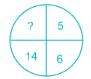Solution:

Here in each column number in the left side = number in the right side × 2 + 2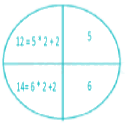Hence the missing number is 12

QUESTION: 18

Directions: Study the following information carefully and answer the questions given below.

Seven boys P, Q, R, S, T, U and V are stand in a straight line facing north as follows.

1. R is standing between S and V.
2. Q is standing to the immediate left of T.
3. V is standing between R and Q.
4. U is Fourth left of V and S is immediate right of P.
Q. Who is standing exactly in the middle?

Solution:

R is standing between S and V
⇒ S       R         V
Q is standing to the immediate left of T.
⇒ Q     T
V is standing between R and Q
⇒ R       V         Q
U is fourth left of V and S is immediate right of P
⇒ U P S R V
Combining all three situations we get,
⇒ U P S R V Q T
R is standing at the middle.

QUESTION: 19

Directions: Study the following information carefully and answer the questions given below.

Seven boys P, Q, R, S, T, U and V are stand in a straight line facing north as follows.

1. R is standing between S and V.
2. Q is standing to the immediate left of T.
3. V is standing between R and Q.
4. U is Fourth left of V and S is immediate right of P.
Q. Who is standing to the extreme left?

Solution:

R is standing between S and V
⇒ S       R         V
Q is standing to the immediate left of T.
⇒ Q     T
V is standing between R and Q
⇒ R       V         Q
U is fourth left of V and S is immediate right of P
⇒ U P S R V
Combining all three situations we get,
⇒ U P S R V Q T
U is standing at the extreme left.

QUESTION: 20

DirectionsIn the following question, you have to identify the correct response from the given premises stated according to following symbols.

Q. If < represent ÷, > represent +, ^ represent –, × represent <, + represent >, - represent= and V represent x

Solution:

As given in information: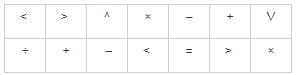1. (8 > 4 < 2 ^ 6 V 3 – 11) = (8 + 4 ÷ 2 – 6 × 3 = 11) = (-8 = 11) which is not true.
2. (8 ^ 4 < 2 > 6 V 3 + 11) = (8 – 4 ÷ 2 + 6 × 3 > 11) = (24 > 11) which is true.
3. (8 V 4 ^ 2 > 6 < 3 × 11) = (8 × 4 – 2 + 6 ÷ 3 < 11) = (32 < 11) which is not true.
4. (8 V 4 > 2 < 6 ^ 3 × 11) = (8 × 4 + 2 ÷ 6 – 3 < 11) = (29.33 <11) which is not true.

Option 2) satisfy the condition.

QUESTION: 21

A square paper is folded in a particular manner and a punch is made. When unfolded, the paper appears as given below.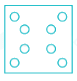Q. Find out the manner in which the paper is folded and punch is made from the responses given

Solution:

Since one dot is present in every corner punch is made in the corner of the square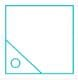QUESTION: 22

Which answer figure will compete the question figure?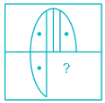Solution:

option figure 4) completes the question figure as: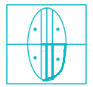QUESTION: 23

How many rectangles are there which are not square in the given figure?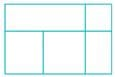Solution:

Numbers of rectangle are shaded below:-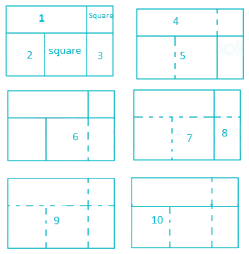Hence the number of rectangles are 10.

QUESTION: 24

In question, one part of the figure is subtracted, choose the option that shows the correct result of subtraction (without the square box):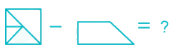Solution:

Given image: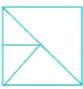If we remove this part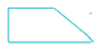We will get such: Dotted lines show removal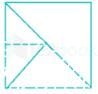Final image (without square box):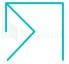Clearly, option 3 is the answer.

QUESTION: 25

If a mirror is placed on the MN line, then which of the answer figures is the right image of the given figure?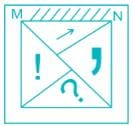Solution:

Here the ‘arrow’ is at the top of the real image. So, it will come at the bottom of the mirror image. And the ‘question’ mark is at the bottom of the real image. So, it will come at the top of the mirror image. Since the mirror is places on the MN line, the ‘exclamation’ mark will remain in the left but its orientation will be opposite. Similar is the case of ‘comma’.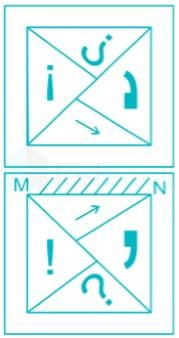Use Code STAYHOME200 and get INR 200 additional OFF Use Coupon Code